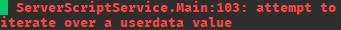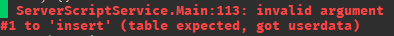# How can I implement the new __iter function to my proxy table?

I have this `proxify` function which allows me to track changes made to my table.

``````local function proxify(tbl: {any})
local proxy = newproxy(true)
local meta = getmetatable(proxy)

meta.__index = function(self, key)
local idx = tbl[key]
if idx and type(idx) == "table" then
idx = proxify(idx)
end
return idx
end

meta.__newindex = function(self, key, newValue)
if tbl[key] ~= newValue then
tbl[key] = newValue
end
end

return proxy
end
``````

However if I try to iterate through it via `for` loop or `table` function, it will throw an error.

``````local myTbl = proxify({
items = {
1, 2, 3, 4, 5
}
})

for _, item in myTbl.items do --> Expected output: 1, 2, 3, 4, 5
print(item)
end
``````That is because the table is no longer just a regular table but a userdata object that acts like a table and allows for tracking changes, etc.

Because the table is now a userdata object, it doesn’t have the `_iter` functionality, and therefore it has to be implemented manually, how can I do that? As of right now I only know this example.

``````metatable.__iter = function(o)
return generator, state, index
end
``````

Source: Syntax - Luau

1 Like

Assuming I understand your question correctly, you’d want to do:

``````metatable.__iter = function()
return next, tbl.items
end
``````

Yes, but how would I implement it? I am just really confused on what generator, state is, and where I suppose to put that function.

If I could, I would have released a module that has complete support of proxies (All services are changed etc…) Sadly it isn’t done yet! I would tell you how to implement it, but it requires you to replace every service for a natural implementation.

If you still wish to learn how I did it then tell me

What do you mean by that?

Yes, I wish to learn, that is the whole point of my post. I just want to be able to iterate through my userdata object like a regular table.

The reason behind me needing a proxy is to track changes so that I can replicate them to client through my module, which I already did make but now I am running into a problem with built-in functions which fail to work on userdata object.

From the example, I believe the `generator` is a function that behaves similarly to the `next` function.

Essentially, it’s a function that you use in order to return a key and value for the loop to receive.

`state` is the table being iterated itself and `index` is the current key the loop is on, or the current key that’s being seen by the `generator` function

Like this?

``````local function customNext()
local key = index + 1
local value = obj[key]
return key, value
end
``````

Can you elaborate more on this?

Yes

What I meant by this is `state` is the table that’s currently being iterated over, like in your proxy example, `state` would be `items` table

So how would I go about implementing the ability to iterate through my userdata object?

``````local myTbl = proxify({
items = {
1, 2, 3, 4, 5
}
})

for _, item in myTbl.items do
print(item)
end
--> Expected output: 1, 2, 3, 4, 5
``````

Where would I put my `__iter` function to begin with? Do I make a function like the `pairs` one?

``````for key, value in customPairs(myTbl.items) do
print(key, value)
end
``````

Apologizes for my lack of knowledge in this area, I didn’t really have any need for metatables and userdata objects until now.

After running a test utilize the code you provided, now I fully see your issue.

What I came up with is doing this inside the `proxify` function:

``````meta.__iter = function()
return next, tbl
end
``````

This would iterate over the table that was provided to the function

I’m not sure if utilizing the `tbl` parameter would be valid for your use case, but it’s the easiest workaround for custom iterating I came up with since iterating over a newproxy isn’t possible

2 Likes

Unfortunately when I do this, I get this.

``````local tbl = proxify({
Timer = 0,
Name = "",
Modes = {
Easy = {},
Normal = {},
Hard = {},
Insane = {}
}
})

for key, value in tbl.Modes do
print(key, value) --> Timer, 0
end
``````

It appears that it’s getting data from the root point of the table. I am pretty sure that we need to use `key` (in the __iter function) to get the right table, as nested tables don’t work.

Hello, I have separated that portion into a model. I will try to be as clear as I can.

What my module does is it will transform proxies, so they look like normal tables. You can use every single service with it and even extend datatypes! You will find a script called ‘Example’.

(We use getfenv() so that it updates the services)

``````local Proxy = require(script.Parent.Proxy)(getfenv());
--By sending the enviroment we have updated all the services

local myProxy = Proxy.new();
``````

The script above creates a proxy.

So now let’s add a metatable! My module adds a custom meta function called “__updated”. This one will fire every time the index already exists but is updated.

``````local myMetatable = {
__newindex = function(self,k,v)
print("This will fire every new index.");
print("The key is", k, "\nAnd the value is", v);
rawset(self,k,v);

print("-----") --Just a break so that the output is separated neatly
end,

__updated = function(self,k,v)
print("This will fire every index that exists and is updated")
print("The key is", k, "\nAnd the value is", v);
rawset(self,k,v);

print("-----") --Just a break so that the output is separated neatly
end,

__index = function(self,k)
print("This will fire every index")
print("The key is", k);

print("-----") --Just a break so that the output is separated neatly
return "nice"; --since we return nice then all the indexes will return nice
end,
};

setmetatable(myProxy, myMetatable);
``````

Done. Now you can use pairs, ipairs, rawset, rawget and all of those to act as normal. There is also an extra function that is extending datatypes

Did you ever wish you could extend Color3 so it has more properties? Me neither. But this module let’s you do that.

``````--[[
If you wish to merge different datatypes now you can!!
Proxy.new can take multiple tables at once.
]]

local mySpecialProxy = Proxy.new(Color3.new(), {}); --Since Color3 is passed in first then it will become the
-- main table *Look bellow for the unary operator*

warn("--Special proxy--");
print(mySpecialProxy.R);
mySpecialProxy.Nice = true; -- Nice is a new value so it will put it in the table
mySpecialProxy.R = 255; -- R already exists so it will modify that value

local myPart = Instance.new("Part");

--myPart.Color = mySpecialProxy; // Oh no! This errored, this is because the proxy is still a table

--If you wish to get the color itself you can use the unary operator

myPart.Color = -mySpecialProxy; --This works
print(myPart.Color);
``````

You can also extend as many datatypes as you wish

``````--// You can also do this
warn("--Extra Special proxy--");
local myExtraSpecialProxy = Proxy.new(Color3.new(), CFrame.new(), {});

print(myExtraSpecialProxy.Position); --Prints the Position
print(myExtraSpecialProxy.R); --Prints R
print(-myExtraSpecialProxy); -- Prints the Color3
``````

Model:
Example.rbxm (6.4 KB)

But mainly you literally only do this:

``````
local myTbl = Proxy.new({
items = {
1, 2, 3, 4, 5
}
});

for _, item in myTbl.items do --> Expected output: 1, 2, 3, 4, 5
print(item)
end``````
2 Likes

Strange, when I run the code on Demo - Luau, I get the tables as results:

``````local function proxify(tbl: {any})
local proxy = newproxy(true)
local meta = getmetatable(proxy)

meta.__index = function(self, key)
local idx = tbl[key]
if idx and type(idx) == "table" then
idx = proxify(idx)
end
return idx
end

meta.__newindex = function(self, key, newValue)
if tbl[key] ~= newValue then
tbl[key] = newValue
end
end

meta.__iter = function()
return next, tbl
end

return proxy
end

local tbl = proxify({
Timer = 0,
Name = "",
Modes = {
Easy = {},
Normal = {},
Hard = {},
Insane = {}
}
})

for key, value in tbl.Modes do
print(key, value)
end
``````

There might be an issue between the site and the Roblox engine, but I can’t see for sure until I get access to my computer again tomorrow

My fault! I had put the wrong table into the `__iter` function. But now I am facing the issue when attempting to use `table.insert` function on the `tbl`.It looks like the `tbl` is returning `userdata` which `table` function can’t handle, how would I go about fixing this issue? I suppose it has to do with `__index`?

Thank you, I will look into your module later, but it sounds great already.

2 Likes

A temporary workaround I did was creating another table inside the `proxify` function and detecting when a key from that table is being indexed inside `tbl` and returning the value associated with the key:

``````local function proxify(tbl: {any})
local proxy = newproxy(true)
local meta = getmetatable(proxy)

local internalMethods = {
insert = function(key, value)
table.insert(tbl, (key or (#tbl + 1)), value)
end
}

meta.__index = function(self, key)
local idx = tbl[key] or internalMethods[key]
if idx and type(idx) == "table" then
idx = proxify(idx)
end
return idx
end

meta.__newindex = function(self, key, newValue)
if tbl[key] ~= newValue then
tbl[key] = newValue
end
end

meta.__iter = function()
return next, tbl
end

return proxy
end

local tbl = proxify({
Timer = 0,
Name = "",
Modes = {
Easy = {},
Normal = {},
Hard = {},
Insane = {}
}
})

tbl.insert(nil, "test")
print(tbl) --> Should print "test"

for key, value in tbl.Modes do
print(key, value)
end
``````

Is it possible to detect when `table` function is accessing the contents of the table?

Another thing is that in the proxy you can see another 2 functions:

1. pmappend. Will append the main table and push the last main table:
``````local Proxy = require(script.Parent.Proxy)(getfenv());
--By sending the enviroment we have updated all the proxies

local myProxy = Proxy.new(CFrame.new());

print(-myProxy); --Prints the CFrame
Proxy.pmappend(myProxy, Color3.new());

print(-myProxy); --Prints the Color
``````
1. pappend. This appends new tables. So you can do:
``````local Proxy = require(script.Parent.Proxy)(getfenv());
--By sending the enviroment we have updated all the proxies

local myProxy = Proxy.new();

Proxy.pappend(myProxy, Color3.new());

print(myProxy.R); --Prints since we appended Color3
``````

If I remember correctly, problem with `getfenv` is that it disables Lua optimizations and I would not want that to happen.

Sorry, I don’t I’m fully understanding, can you elaborate? Are you asking is there a way to dynamically connect the functions for the `table` global, to the proxy table? If so, I believe there is